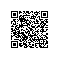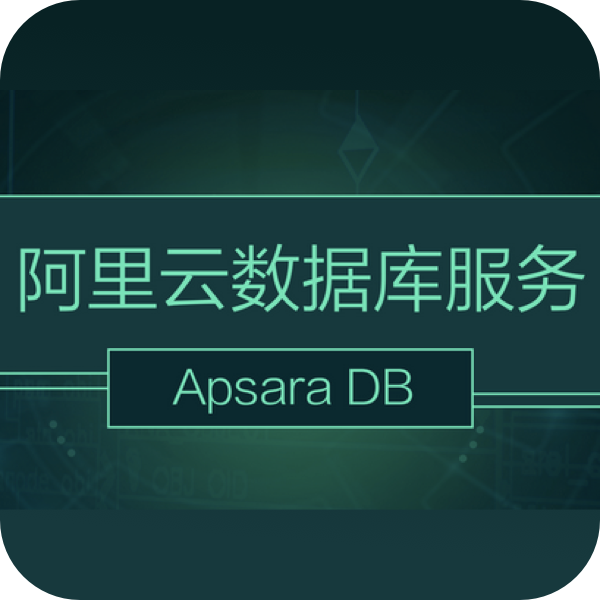# PostgreSQL cube 插件 - 多维空间对象

### 标签

PostgreSQL , cube , GiST索引 , 多维 , 欧几里得

## 背景

CUBE是一个多维数据类型，支持两种多维类型：多维POINT、区间（左下+右上）。以及这些几何对象的几何特性搜索和计算（方位搜索、距离计算），这些搜索都支持GiST索引。## 语法

External Syntax Meaning
x 点，A one-dimensional point (or, zero-length one-dimensional interval)
(x) Same as above
x1,x2,...,xn 点，A point in n-dimensional space, represented internally as a zero-volume cube
(x1,x2,...,xn) Same as above
(x),(y) 一维区间（线段）（两个括号隔开，分别表示每个维度的最小值(左括号)和最大值(右括号)），A one-dimensional interval starting at x and ending at y or vice versa; the order does not matter
[(x),(y)] Same as above
(x1,...,xn),(y1,...,yn) 多维区间（平面方形、立方体、多维CUBE）（两个括号隔开，分别表示每个维度的最小值(左括号)和最大值(右括号)）An n-dimensional cube represented by a pair of its diagonally opposite corners
[(x1,...,xn),(y1,...,yn)] Same as above

## 操作符

Operator Result Description
a = b boolean The cubes a and b are identical.
a && b boolean The cubes a and b overlap.
a @> b boolean The cube a contains the cube b.
a <@ b boolean The cube a is contained in the cube b.
a < b boolean The cube a is less than the cube b.
a <= b boolean The cube a is less than or equal to the cube b.
a > b boolean The cube a is greater than the cube b.
a >= b boolean The cube a is greater than or equal to the cube b.
a <> b boolean The cube a is not equal to the cube b.
a -> n float8 Get n-th coordinate(坐标) of cube (counting from 1).
a ~> n float8 Get n-th coordinate in “normalized” cube representation, in which the coordinates have been rearranged into the form “lower left — upper right”; that is, the smaller endpoint along each dimension appears first.
a <-> b float8 欧几里得距离。Euclidean distance between a and b.
a <#> b float8 分别每个坐标的距离，求和。Taxicab (L-1 metric) distance between a and b.
a <=> b float8 分别每个坐标的距离，取最大值。Chebyshev (L-inf metric) distance between a and b.

## 函数

Function Result Description Example
cube(float8) cube Makes a one dimensional cube with both coordinates the same. cube(1) == '(1)'
cube(float8, float8) cube Makes a one dimensional cube. cube(1,2) == '(1),(2)'
cube(float8[]) cube Makes a zero-volume cube using the coordinates defined by the array. cube(ARRAY[1,2]) == '(1,2)'
cube(float8[], float8[]) cube Makes a cube with upper right and lower left coordinates as defined by the two arrays, which must be of the same length. cube(ARRAY[1,2], ARRAY[3,4]) == '(1,2),(3,4)'
cube(cube, float8) cube Makes a new cube by adding a dimension on to an existing cube, with the same values for both endpoints of the new coordinate. This is useful for building cubes piece by piece from calculated values. cube('(1,2),(3,4)'::cube, 5) == '(1,2,5),(3,4,5)'
cube(cube, float8, float8) cube Makes a new cube by adding a dimension on to an existing cube. This is useful for building cubes piece by piece from calculated values. cube('(1,2),(3,4)'::cube, 5, 6) == '(1,2,5),(3,4,6)'
cube_dim(cube) integer Returns the number of dimensions of the cube. cube_dim('(1,2),(3,4)') == '2'
cube_ll_coord(cube, integer) float8 Returns the n-th coordinate value for the lower left corner of the cube. cube_ll_coord('(1,2),(3,4)', 2) == '2'
cube_ur_coord(cube, integer) float8 Returns the n-th coordinate value for the upper right corner of the cube. cube_ur_coord('(1,2),(3,4)', 2) == '4'
cube_is_point(cube) boolean Returns true if the cube is a point, that is, the two defining corners are the same. -
cube_distance(cube, cube) float8 Returns the distance between two cubes. If both cubes are points, this is the normal distance function. -
cube_subset(cube, integer[]) cube Makes a new cube from an existing cube, using a list of dimension indexes from an array. Can be used to extract the endpoints of a single dimension, or to drop dimensions, or to reorder them as desired. cube_subset(cube('(1,3,5),(6,7,8)'), ARRAY) == '(3),(7)' cube_subset(cube('(1,3,5),(6,7,8)'), ARRAY[3,2,1,1]) == '(5,3,1,1),(8,7,6,6)'
cube_union(cube, cube) cube Produces the union of two cubes. -
cube_inter(cube, cube) cube Produces the intersection of two cubes. -
cube_enlarge(c cube, r double, n integer) cube Increases the size of the cube by the specified radius r in at least n dimensions. If the radius is negative the cube is shrunk instead. All defined dimensions are changed by the radius r. Lower-left coordinates are decreased by r and upper-right coordinates are increased by r. If a lower-left coordinate is increased to more than the corresponding upper-right coordinate (this can only happen when r < 0) than both coordinates are set to their average. If n is greater than the number of defined dimensions and the cube is being enlarged (r > 0), then extra dimensions are added to make n altogether; 0 is used as the initial value for the extra coordinates. This function is useful for creating bounding boxes around a point for searching for nearby points. cube_enlarge('(1,2),(3,4)', 0.5, 3) == '(0.5,1.5,-0.5),(3.5,4.5,0.5)'

## 例子

1、向量聚合（类似多维聚集）

https://github.com/umitanuki/kmeans-postgresql

2、4维（包含）的聚集分析

http://postgis.net/docs/manual-2.3/ST_ClusterKMeans.html

3、求多维点的距离

欧几里得距离。

postgres=# select '(1,2,3,4)'::cube <-> '(2,2,3,10)'::cube ;
?column?
------------------
6.08276253029822
(1 row)

postgres=# select '(1,2,3,4)'::cube <=> '(2,2,3,10)'::cube ;
?column?
----------
6
(1 row)

postgres=# select '(1,2,3,4)'::cube <#> '(2,2,3,10)'::cube ;
?column?
----------
7
(1 row)


4、按距离排序，输出附近的多维点。

SELECT c FROM test ORDER BY c <-> cube(array[0.5,0.5,0.5]) LIMIT 1;


5、假设某个表有多个数值类型字段，基于这几个字段构建CUBE，创建gist表达式索引。将来可以根据这个按距离高速检索附近的多维点（记录）。

postgres=# create index idx on tbl_tmp using gist (cube(array[c1,c3,c4,c5]));


6、针对以上索引，我们可以对数据进行聚集存储，实现高效过滤。

create table tbl(c1 int, c2 int, c3 numeric, c4 float4, c5 int, c6 int);

insert into tbl select random()*1000, random()*1000000, random()*100000000, random()*100000, random()*1000000, random()*100 from generate_series(1,10000000);

create index idx_tbl_1 on tbl using gist(cube(array[c1::float8,c2::float8,c3::float8,c4::float8,c5::float8,c6::float8]));

create index idx_tbl_2 on tbl using brin(c1,c2,c3,c4,c5,c6);

postgres=# explain (analyze,verbose,timing,costs,buffers) select * from tbl where c5 between 1 and 10  and c4 between 1 and 5;
QUERY PLAN
------------------------------------------------------------------------------------------------------------------------------------
Bitmap Heap Scan on public.tbl  (cost=8.51..218370.51 rows=1 width=31) (actual time=596.689..596.689 rows=0 loops=1)
Output: c1, c2, c3, c4, c5, c6
Recheck Cond: ((tbl.c4 >= '1'::double precision) AND (tbl.c4 <= '5'::double precision) AND (tbl.c5 >= 1) AND (tbl.c5 <= 10))
Rows Removed by Index Recheck: 4980743
Heap Blocks: lossy=9146
Buffers: shared hit=9152
->  Bitmap Index Scan on idx_tbl_2  (cost=0.00..8.51 rows=10000000 width=0) (actual time=0.229..0.229 rows=92160 loops=1)
Index Cond: ((tbl.c4 >= '1'::double precision) AND (tbl.c4 <= '5'::double precision) AND (tbl.c5 >= 1) AND (tbl.c5 <= 10))
Buffers: shared hit=6
Planning time: 0.126 ms
Execution time: 596.727 ms
(11 rows)

postgres=# explain (analyze,verbose,timing,costs,buffers) select * from tbl where c5 between 1 and 10  and c6 between 1 and 5;
QUERY PLAN
----------------------------------------------------------------------------------------------------------------------------
Bitmap Heap Scan on public.tbl  (cost=6.25..120154.09 rows=1 width=31) (actual time=106.353..609.540 rows=2 loops=1)
Output: c1, c2, c3, c4, c5, c6
Recheck Cond: ((tbl.c5 >= 1) AND (tbl.c5 <= 10) AND (tbl.c6 >= 1) AND (tbl.c6 <= 5))
Rows Removed by Index Recheck: 5399033
Heap Blocks: lossy=9914
Buffers: shared hit=9916
->  Bitmap Index Scan on idx_tbl_2  (cost=0.00..6.25 rows=5089292 width=0) (actual time=0.207..0.207 rows=99840 loops=1)
Index Cond: ((tbl.c5 >= 1) AND (tbl.c5 <= 10) AND (tbl.c6 >= 1) AND (tbl.c6 <= 5))
Buffers: shared hit=2
Planning time: 0.113 ms
Execution time: 609.588 ms
(11 rows)


postgres=# cluster tbl USING idx_tbl_1;


postgres=# explain (analyze,verbose,timing,costs,buffers) select * from tbl where c5 between 1 and 10  and c4 between 1 and 5;
QUERY PLAN
------------------------------------------------------------------------------------------------------------------------------------
Bitmap Heap Scan on public.tbl  (cost=8.51..218375.51 rows=1 width=31) (actual time=219.648..219.648 rows=0 loops=1)
Output: c1, c2, c3, c4, c5, c6
Recheck Cond: ((tbl.c4 >= '1'::double precision) AND (tbl.c4 <= '5'::double precision) AND (tbl.c5 >= 1) AND (tbl.c5 <= 10))
Rows Removed by Index Recheck: 1881220
Heap Blocks: lossy=3456
Buffers: shared hit=3458
->  Bitmap Index Scan on idx_tbl_2  (cost=0.00..8.51 rows=10000000 width=0) (actual time=0.133..0.133 rows=34560 loops=1)
Index Cond: ((tbl.c4 >= '1'::double precision) AND (tbl.c4 <= '5'::double precision) AND (tbl.c5 >= 1) AND (tbl.c5 <= 10))
Buffers: shared hit=2
Planning time: 0.134 ms
Execution time: 219.685 ms
(11 rows)

postgres=# explain (analyze,verbose,timing,costs,buffers) select * from tbl where c5 between 1 and 10  and c6 between 1 and 5;
QUERY PLAN
----------------------------------------------------------------------------------------------------------------------------
Bitmap Heap Scan on public.tbl  (cost=6.25..120159.09 rows=1 width=31) (actual time=43.253..315.421 rows=2 loops=1)
Output: c1, c2, c3, c4, c5, c6
Recheck Cond: ((tbl.c5 >= 1) AND (tbl.c5 <= 10) AND (tbl.c6 >= 1) AND (tbl.c6 <= 5))
Rows Removed by Index Recheck: 2857135
Heap Blocks: lossy=5248
Buffers: shared hit=5250
->  Bitmap Index Scan on idx_tbl_2  (cost=0.00..6.25 rows=5089292 width=0) (actual time=0.147..0.147 rows=52480 loops=1)
Index Cond: ((tbl.c5 >= 1) AND (tbl.c5 <= 10) AND (tbl.c6 >= 1) AND (tbl.c6 <= 5))
Buffers: shared hit=2
Planning time: 0.111 ms
Execution time: 315.462 ms
(11 rows)


## 参考

https://www.postgresql.org/docs/10/static/cube.html

http://postgis.net/docs/manual-2.3/ST_ClusterKMeans.html

https://github.com/umitanuki/kmeans-postgresql使用钉钉扫一扫加入圈子
+ 订阅